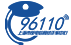﻿ 学校频道 - 东方财富网

• 股票
• 期货
• 外汇
• 固收
• 理财
• 保险
• 科创板

• 股票
• 期货
• 外汇
• 固收
• 理财
• 保险

• 股票
• 期货
• 外汇
• 固收
• 理财
• 保险

• 与企业经营有关的资产占总资产的比例越高越好。我们在选择公司的时候，最优秀的公司，与企业经营有关的资产占总资产的比例>90%，一般来说，一家好公司最起码也应该>70%。通过分析投资类资产，可以了解一家公司的主业专注度。在实践中，与主业无关的投资类资产占总资产比率大于20%的公司，至少表明其不够专注，在...
03-17 10:49
• 全面注册制下，不仅公司上市流程会产生改变，股民交易的生态也会发生变化。快来看看其中涉及到的具体交易规则和解读吧！
03-16 13:26
• 对于企业所投入的隐性成本，经济利润会考虑在别处能挣到什么？而会计利润则不考虑隐性成本，不列入利润表的减项。理由是：没有客观的办法计算隐性成本，会计师不愿意做没有根据的估计。但作为一个企业所有者其实不仅仅关心会计利润，而且也关心经济利润。
03-08 11:23
• 全面注册制在主板引入“价格笼子”(即“有效申报价格范围”)，同时平移了科创板的“拒单模式”，即超出有效价格范围的订单将被系统拒收成为“废单”，主板交易制度的这一重大变化，让很多打板一族不适应。
03-06 13:32

• 热门
• 房地产
• 银行
• 券商信托
• 工程建设
• 水泥建材
• 家电行业
• 电子信息
• 汽车行业
• 化工行业
• 医药制造

• B
• C
• D
• F
• G
• H
• J
• K
• L
• M
• N
• P
• Q
• R
• S
• T
• W
• X
• Y
• Z
• B
• C
• G
• H
• J
• M
• N
• P
• S
• W
• X
• Z
• A
• C
• D
• F
• G
• H
• N
• S
• T
• X
• Z
• A
• B
• C
• D
• G
• H
• J
• K
• L
• N
• P
• Q
• S
• T
• W
• X
• Y
• Z
• B
• D
• F
• G
• H
• J
• K
• L
• N
• Q
• S
• T
• W
• X
• Y
• Z
• A
• B
• C
• D
• F
• G
• H
• J
• K
• L
• M
• Q
• R
• S
• T
• W
• X
• Y
• Z
• A
• B
• C
• D
• E
• F
• G
• H
• J
• K
• L
• M
• P
• R
• S
• T
• X
• Y
• Z
• A
• B
• C
• D
• F
• G
• H
• J
• K
• L
• M
• N
• Q
• R
• S
• T
• W
• X
• Y
• Z
• A
• B
• C
• D
• F
• G
• H
• J
• K
• L
• M
• N
• P
• Q
• R
• S
• T
• W
• X
• Y
• Z
• A
• B
• C
• D
• E
• F
• G
• H
• J
• K
• L
• M
• N
• P
• Q
• R
• S
• T
• W
• X
• Y
• Z